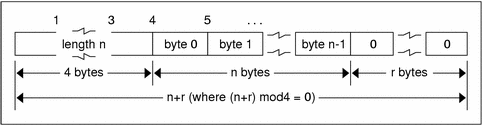ONC+ Developer's Guide

## Variable-Length Opaque Data

The standard also provides for variable-length counted opaque data. Such data is defined as a sequence of n (numbered 0 through n-1) arbitrary bytes to be the number n encoded as an unsigned integer, as described subsequently, and followed by the n bytes of the sequence.

Byte b of the sequence always precedes byte b+1 of the sequence, and byte 0 of the sequence always follows the sequence's length. The n bytes are followed by enough (0 to 3) residual zero bytes, r, to make the total byte count a multiple of four.

### Declaration

Variable-length opaque data is declared in the following way.

`opaque identifier<m>; `

or

`opaque identifier<>;; `

The constant m denotes an upper bound of the number of bytes that the sequence can contain. If m is not specified, as in the second declaration, it is assumed to be (2**32) - 1, the maximum length. For example, a filing protocol might state that the maximum data transfer size is 8192 bytes, as follows.

`opaque filedata<8192>;`

### Variable-Length Opaque EncodingDo not encode a length greater than the maximum described in the specification.